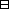## Group Theory and Linear Algebra

Last updated: 16 September 2014

## Lecture 2: gcd and Euclid's algorithm

### Number systems – $ℤ,$ the integers

$ℤ= { …,(-1)+ (-1)+(-1), (-1)+(-1), -1,0,1,1+1,1 +1+1,… }$ with $\left(-1\right)+1=0,$ $1+\left(-1\right)=0,$ $0+1=1,$ $1+0=1,$ $0+\left(-1\right)=-1,$ $\left(-1\right)+0=-1\text{.}$

Let $d\in ℤ\text{.}$ The multiples of $d$ is $dℤ= { …,(-d)+ (-d)+(-d), (-d)+(-d), -d,0,d,d+d,d +d+d,… } .$

Let $a,d\in ℤ\text{.}$ The integer $d$ divides $a,$ $d|a,$ if $a\in dℤ\text{.}$

Let $x,m\in ℤ\text{.}$ The greatest common divisor of $x$ and $m,$ $\text{gcd}\left(x,m\right),$ is $d\in {ℤ}_{>0}$ such that

 (a) $d|x$ and $d|m,$ (b) If $\ell \in {ℤ}_{>0}$ and $\ell |x$ and $\ell |m$ then $\ell |d\text{.}$

Let $a,b\in ℤ\text{.}$ Define $a if there exists $x\in {ℤ}_{>0}$ such that $a+x=b\text{;}$
$a\le b$ if $a or $a=b\text{.}$

(Euclidean algorithm) Let $a,b\in ℤ\text{.}$ There exist unique $q,r\in ℤ$ such that

 (a) $a=bq+r,$ (b) $0\le r<|b|,$ where $|b|=\left\{\begin{array}{cc}b,& \text{if} b\in {ℤ}_{>0},\\ 0,& \text{if} b=0,\\ -b,& \text{if} -b\in {ℤ}_{>0}\text{.}\end{array}$

If (a) and (b) hold write $a=r \text{mod} b\text{.}$

The 15th row of the multiplication table for $ℤ}{36ℤ}$ is $· 1 2 3 4 5 6 7 8 9 10 11 12 13 14 15 16 17 18 19 ⋯ 15 15 30 9 24 3 18 33 12 27 6 21 36 15 30 9 24 3 ⋯$ Notice that

 (a) $15·10 = 150inℤ, 150 = 4·36+6,and 15·10 = 6inℤ36ℤ.$ (b) The numbers in row $15$ of the multiplication table for $ℤ}{36ℤ}$ are $3,6,9,12,15,18,21, 24,27,30,33,36$ (all multiples of $3$ in $ℤ}{36ℤ}\text{).}$ (c) $3=15·17+12\left(-7\right)\text{.}$

Let $x,m\in ℤ\text{.}$ There exists $\ell \in {ℤ}_{>0}$ such that $ℓℤ=xℤ+mℤ.$

Let $x,m\in ℤ\text{.}$ Let $\ell \in {ℤ}_{>0}$ such that $\ell ℤ=xℤ+mℤ\text{.}$ Let $g=\text{gcd}\left(x,m\right)\text{.}$ Then $d=\ell \text{.}$

(Euclidean algorithm). Let $a,b\in ℤ\text{.}$ There exist unique $q,r\in ℤ$ such that

 (a) $a=bq+r,$ (b) $0\le r<|b|,$ where $|b|=\left\{\begin{array}{cc}b,& \text{if} b\in {ℤ}_{>0},\\ 0,& \text{if} b=0,\\ -b,& \text{if} -b\in {ℤ}_{>0}\text{.}\end{array}$Proof.

Assume $a,b\in ℤ\text{.}$
To show:
(a) There exist $q,r\in ℤ$ such that
 (1) $a=bq+r,$ (2) $0\le r<|b|\text{.}$
(b) $q,r\in ℤ$ such that $a=bq+r$ and $0\le r<|b|$ are unique.
(a) Let $bq=$ the smallest integer in $bℤ$ less than or equal to $a$ and $r=a-qb\text{.}$
To show:
 (aa) $a=bq+r,$ (ab) $0\le r<|b|\text{.}$
 (aa) Since $r=a-qb$ then $a=bq+r\text{.}$ (ab) Since $bq\le a$ and $b\left(q+1\right)>a,$ $0\le a-bq$ and $b>a-bq\text{.}$ So $0\le r$ and $b>r\text{.}$
(b) Assume ${q}_{1},{r}_{1},\in ℤ$ and $a=b{q}_{1}+{r}_{1}$ and $0\le {r}_{1}<|b|$ and assume ${q}_{2},{r}_{2}\in ℤ$ and $a=b{q}_{2}+{r}_{2}$ and $0\le {r}_{2}<|b|\text{.}$
${q}_{1}={q}_{2}$ and ${r}_{1}={r}_{2}\text{.}$
Since $a-{r}_{1}=b{q}_{1}$ and $0\le {r}_{1}<|b|,$ $b{q}_{1}$ is the largest integer in $bℤ$ which is $\le a\text{.}$
Since $a-{r}_{2}=b{q}_{2}$ and $0\le {r}_{2}<|b|,$ $b{q}_{2}$ is the largest integer in $bℤ$ which is $\le a\text{.}$
So $b{q}_{1}=b{q}_{2}$ and ${q}_{1}={q}_{2}\text{.}$
So ${r}_{1}=a-b{q}_{1}=a-b{q}_{2}={r}_{2}\text{.}$

$\square$

Using Euclid's algorithm find $gcd(1288,1144).$ Hodgson says: If $a=bq+r$ with $0\le r<|b|$ then $\text{gcd}\left(a,b\right)=\text{gcd}\left(b,r\right)\text{.}$ $1288 = 1144+144 1144 = 7·144+136 144 = 136+8 136 = 17·8+0 9·144 = 1296 8·144 = 1152 7·144 = 1008$ So $gcd(1288,144) = gcd(1144,144) = gcd(144,136) = gcd(136,8) = gcd(8,0)=8.$ Note: $8 = 144-136 = 144-(1144-7·144)= 8·144-1144 = 8(1288-1144)-1144 = 8·1288-9·1144.$

Let $x,m\in {ℤ}_{>0}$ with $1\le x\le m\text{.}$ There exists $\ell \in {ℤ}_{>0}$ such that $ℓℤ=xℤ+mℤ.$Proof.

Let $\ell \in {ℤ}_{>0}$ be minimal such that $\ell \in xℤ+mℤ\text{.}$
To show: $\ell ℤ=zℤ+mℤ\text{.}$
To show:
 (a) $\ell ℤ\subseteq xℤ+mℤ\text{.}$ (b) $xℤ+mℤ\subseteq \ell ℤ\text{.}$
 (a) Since $\ell \in xℤ+mℤ,$ $ℓℤ⊆xℤ+mℤ.$ (b) Assume $y\in xℤ+mℤ\text{.}$ To show: $y\in \ell ℤ\text{.}$ Since $\ell$ is minimal $y\ge \ell \text{.}$ So $y=q\ell +r$ with $0\le r<\ell \text{.}$ So $r=y-q\ell \in xℤ+mℤ\text{.}$ So $r=0,$ since $\ell$ is minimal positive integer in $xℤ+mℤ\text{.}$ So $y=q\ell \text{.}$ So $y\in \ell ℤ\text{.}$ So $\ell ℤ=xℤ+mℤ\text{.}$

$\square$

Let $x,m\in ℤ\text{.}$ Let $\ell \in {ℤ}_{>0}$ such that $\ell ℤ=xℤ+mℤ\text{.}$ Let $d=\text{gcd}\left(x,m\right)\text{.}$ Then $d=\ell \text{.}$Proof.

Let $d=\text{gcd}\left(x,m\right)\text{.}$
Let $\ell \in {ℤ}_{>0}$ such that $\ell ℤ=xℤ+mℤ\text{.}$
To show: $\ell =d\text{.}$
To show:
 (a) $\ell |d\text{.}$ (b) $d|\ell \text{.}$
 (a) Since $x\in \ell ℤ,$ then $\ell |x\text{.}$ Since $m\in \ell ℤ,$ then $\ell |m\text{.}$ Since $d=\text{gcd}\left(x,m\right),$ then $\ell |d\text{.}$ (b) Since $d|x$ and $d|m,$ then $x\in dℤ$ and $m\in dℤ\text{.}$ So $xℤ+mℤ\subseteq dℤ\text{.}$ So $\ell ℤ\subseteq dℤ\text{.}$ So $\ell \in dℤ\text{.}$ So $d|\ell \text{.}$

$\square$

## Notes and References

These are a typed copy of Lecture 2 from a series of handwritten lecture notes for the class Group Theory and Linear Algebra given on July 27, 2011.